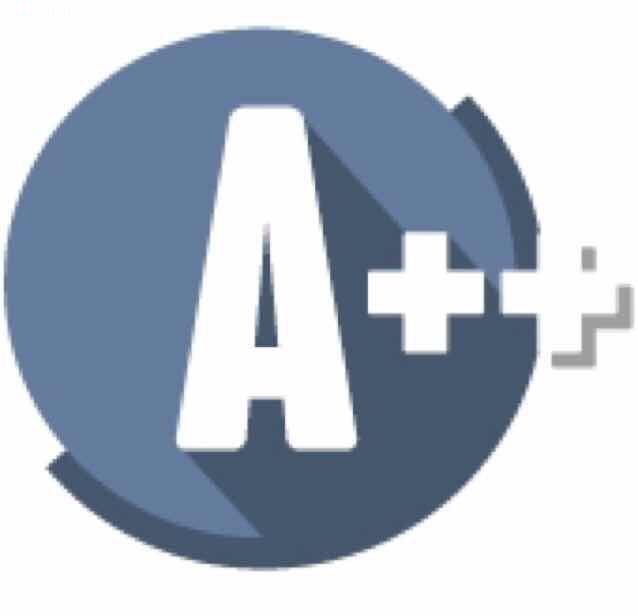## Interviews

#### Number of Subarrays with Bounded Maximum# LeetCode 795 Number of Subarrays with Bounded Maximum

We are given an array A of positive integers, and two positive integers L and R (L <= R).
Return the number of (contiguous, non-empty) subarrays such that the value of the maximum array element in that subarray is at least L and at most R.

#### Example :

Input:
A = [2, 1, 4, 3]
L = 2
R = 3
Output: 3
Explanation: There are three subarrays that meet the requirements: , [2, 1], .
Note:
* L, R  and A[i] will be an integer in the range [0, 10^9].
* The length of A will be in the range of [1, 50000].

``````
public int numSubarrayBoundedMax(int[] A, int L, int R) {
int pre = -1;
int count = 0;

for(int i = 0; i < A.length; i++){
if(A[i] >=  L && A[i] <= R){
count += i - head + 1;
pre = i;
}
else if(A[i] <  L){
if(pre != -1){
count += pre - head + 1;
}
}
else if(A[i] > R) {
pre = -1;
}
}
return count;
}
``````